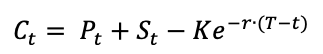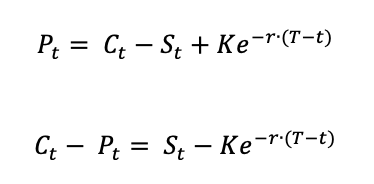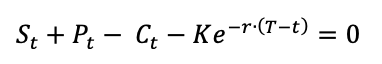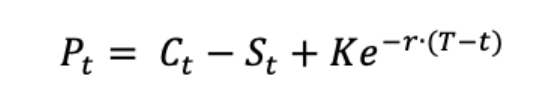Call-Put ParityThis article written by Akshit GUPTA (ESSEC Business School, Master in Management, 2019-2022) presents the subject of call-put parity.

Introduction

The call-put parity (also written the put-call parity) is a concept introduced in the 1960s by the economist Hans R. Stoll in a paper named “The Relationship Between Put and Call Option Prices”. The call-put parity shows the relationship between the prices of a put option, a call option, and the underlying asset. The call option and the put option are written on the same underlying asset and have the same expiration date and strike price. The call-put parity is applicable only on European options with a fixed time to expiration (it is not applicable to American options).

Call-put parity relation

The call-put parity relation is given by the equality:Where t is the evaluation date (any date between the issuance date and the maturity date of the option), Ct the price of the call option, Pt the price of the put option, St the price of the underlying asset, K the strike price of the two options (same strike price for the call and put options), T the maturity date of the two options (same maturity date for the call and put options) and r the risk-free rate.

The call-put parity relation is sometimes written in different ways:Demonstration

Let us try to find the call-put parity relation for a put option and a call option, which are European options with the same strike price K and the same maturity date T.

Let us consider a portfolio composed a long position in the underlying asset, a long position in the put option, a short position in the call option and a short position of a zero-coupon bond maturing at time T and of final value K.

Let us compute the value of this position at time T. The underlying asset is worth ST. The zero-coupon bond is worth K. Regarding the call and put options, we can distinguish two cases: ST > K and ST < K.

In the first case, the put option finishes out of the money and the call finishes in the money and is worth STK. The value of the position is then equal to: ST + 0 – (STK) – K, which is equal to zero.

In the second case, the call option finishes out of the money and the put finishes in the money and is worth K – ST. The value of the position is then equal to: ST + (KST) – 0 – K, which is equal to zero.

If the value of the position at time T is also equal to 0, then the value of the position at time t is also equal to 0. If there is no arbitrage, then the value of the position by detailing its components satisfies:which leads to the formula given above.

Application

The call-put parity formula helps the investors to calculate the price of a put option from the price of a call option, or inversely, to calculate the price of a call option from the price of a put option (the call option and the put option are written on the same underlying asset and have the same expiration date T and strike price K).

Implication

If the put-call parity does not hold true, there exists an arbitrage opportunity for investors. An arbitrage opportunity helps the investors earn profits without taking any risks. But the chances of finding an arbitrage opportunity is low given the high liquidity in the markets.

Example of application of the call-put parity

Assuming the stock of APPLE is trading at \$25 in the market, the strike price of a 3-month European call option on Apple stock is \$24 and the premium is \$5. The risk-free rate is 8%.

Now, using the call-put parity,we can calculate the price of the 3-month European put option on Apple stock with the same strike price, which is as follows:

The price of the call option (C) is \$5, the price of the underlying asset (S) is \$25, the present value of the strike price (K) is \$23.52, and the risk-free rate (r) is 8% (market data).

As per the formula: P = \$5 – \$25 + \$23.52, the price of the put option (P) is approximately equal to \$3.52.

Related posts

Gupta A. History of Options markets
Gupta A. Option Trader – Job description
Gupta A. Options

Useful resources

Hull J.C. (2015) Options, Futures, and Other Derivatives, Ninth Edition, Chapter 11 – Properties of Stock Options, 256-275.

Stoll H.R. (1969) “The Relationship Between Put and Call Option Prices,” The Journal of Finance, 24(5): 801-824.